# DAV Class 8 Maths Chapter 1 Worksheet 4 Solutions

The DAV Class 8 Maths Book Solutions and DAV Class 8 Maths Chapter 1 Worksheet 4 Solutions of Squares and Square Roots offer comprehensive answers to textbook questions.

## DAV Class 8 Maths Ch 1 WS 4 Solutions

Question 1.
Find the square root of the following numbers by the long division method.
(i) 9801
(ii) 6561
(iii) 390625
(iv) 108241
(v) 363609
(vi) 120409
(vii) 1471369
(viii) 57121.
Solution:
(i) The given number is 9801.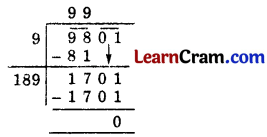Hence $$\sqrt{9801}$$ = 99.

(ii) The given number is 6561.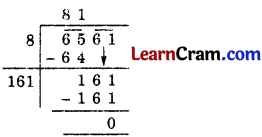Hence $$\sqrt{6561}$$ = 81.

(iii) The given number is 390625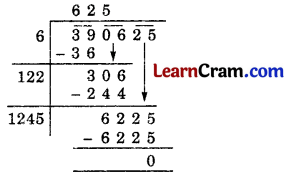Hence $$\sqrt{390625}$$ = 625.(iv) The given number is 108241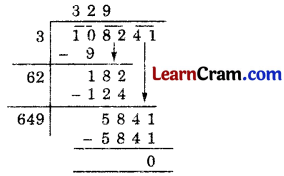Hence $$\sqrt{108241}$$ = 329.

(v) The given number is 363609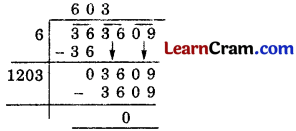Hence $$\sqrt{363609}$$ = 603.

(vi) The given number is 120409Hence $$\sqrt{120409}$$ = 347.

(vii) The given number is 1471369Hence $$\sqrt{1471369}$$ = 1213.

(viii) The given number is 57121Hence $$\sqrt{57121}$$ = 239.Question 2.
Find the least number which must be subtracted from 6203 to obtain a perfect square. Also, find the square root of the number so obtained.
Solution:
The given number is 6203.
∴ 119 must be subtracted from 6203 to obtain a perfect square.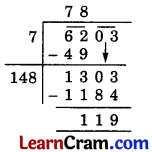Hence the perfect square number is 6203 – 119 = 6084
and $$\sqrt{6084}$$ = 78.

Question 3.
Find the greatest number of six digits which is a prefect square. Find the square root of this number.
Solution:
The greatest 6-digit number is 999999.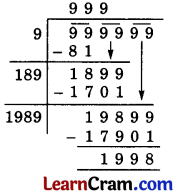So, the greatest 6-digit square number is = 999999 – 1998 = 998001
and $$\sqrt{998001}$$ =999Question 4.
Find the least number which must be added to 6203 to obtain a prefect square. Also, find the square root of the number so obtained.
Solution:
The given number is 6203.
So, 61 is the least number which when added to the given number 6023, it becomes a perfect square.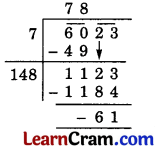∴ Perfect square number is 6023 + 61 = 6084
and $$\sqrt{6084}$$ = 78.

Question 5.
Find the least number of six digits which is a perfect square. Find the square root of this number.
Solution:
Let the least six-digit number be 100000.
But remainder (+) iveSo we will try again to get a negative remainder Hence 489 must be added to the given number to obtain a least perfect square of six-digit.
∴ The required number = 100000 + 489 = 100489
and $$\sqrt{100459}$$ = 317.Question 6.
Find the value of $$\sqrt{64432729}$$ – $$\sqrt{9653449}$$.
Solution:
Apply long division method to find the square root of 64432729 and 9653449.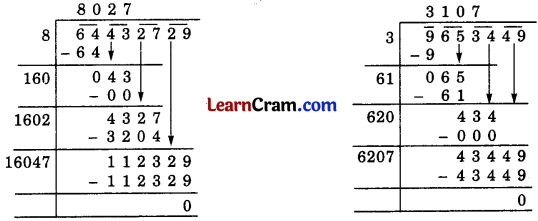Thus, the value of $$\sqrt{64432729}$$ – $$\sqrt{9653449}$$ = 8027 – 3107 = 4920.Question 1.
Find the square root of the following numbers by long division method.
(i) 1521
(ii) 4401604
(iii) 99856
(iv) 3249
Solution:
(i) 1521Hence $$\sqrt{1521}$$ = 39.

(ii) 4401604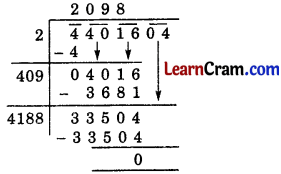Hence $$\sqrt{4401604}$$ = 2098

(iii) 99856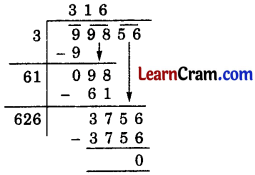Hence $$\sqrt{99856}$$ = 316

(iv) 3249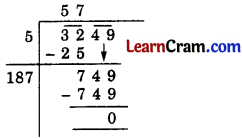Hence $$\sqrt{3249}$$ = 57.Question 2.
Find the number of digits in the square root of each of the following numbers (without calculation).
(i) 81
(ii) 225
(iii) 4489
(iv) 27225
Solution:
(i) Number of digit ‘n’ in 81 is 2 (even).
∴ Number of digits in its square root = $$\frac{n}{2}=\frac{2}{2}$$ = 1
Hence, the square root of 81 has 1 digit.

(ii) Number of digits ‘n’ in 225 is 3 (odd).
∴ Number of digits in its square root = $$\frac{n+1}{2}=\frac{3+1}{2}$$ = 2
Hence, the square root of 225 has 2 digits.

(iii) Number of digits ‘n’ in 4489 is 4 (even).
∴ Number of digits in its square root = $$\frac{n}{2}=\frac{4}{2}$$ = 2.
Hence, the number of digits in its square root is 2.

(iv) Number of digits ‘n’ in 27225 is 5 (odd).
∴ Number of digits in its square root = $$\frac{n+1}{2}=\frac{5+1}{2}$$ = 3
Hence, the square root of 27225 has 3 digits.Question 3.
Find the least number which must be subtracted from 7581 to obtain a perfect square. Find this perfect square number and its square root.
Solution:∴ 12 should be subtracted from 7581 to get a prefect square number.
The perfect square number = 7581 – 12= 7569
Hence, $$\sqrt{7569}$$ = 87

Question 4.
Find the least number which must be added to 6412 to obtain a perfect square number. Find this number and its square root.
Solution: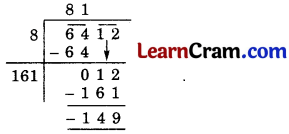So, 149 should be added to 6412 to get a perfect square number.
The perfect square number = 6412 + 149 = 6561
and $$\sqrt{6561}$$ = 81

Question 5.
The area of a field is 1825 m2. What area should be added or subtracted to obtain a square field? Also, find this area and the side of the square field.
Solution: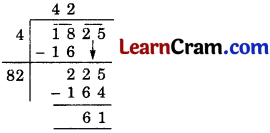Case I:
So, 61 m2 should be subtracted from the area 1825 m2 to get a square field.
∴ The area of this square field = 1825 – 61 = 1764
and the side of this field = $$\sqrt{1764}$$ = 42 m

Case II:
So, 24 m2 should be added to the area 1825 m2 to get a square field.
∴ The area of the square field = 1825 + 24 = 1849 m2and the side of this field = $$\sqrt{1849}$$ = 43 m.Question 6.
Find the length of the side of a square field of area 7744 m2. Also, find its perimeter.
Solution:
Let the side of the square be x m.
Its area = x2 m2
∴ x2 = 7744
∴ x = $$\sqrt{7744}$$ = 88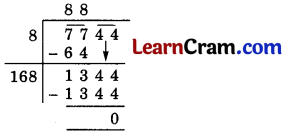Hence, the length of the side of the given square = 88 m
and its perimeter = 4 × side = 4 × 88 = 352 m